Courses

# JEE Main Physics Mock - 2

## 25 Questions MCQ Test Mock Test Series for JEE Main & Advanced 2021 | JEE Main Physics Mock - 2

Description
This mock test of JEE Main Physics Mock - 2 for JEE helps you for every JEE entrance exam. This contains 25 Multiple Choice Questions for JEE JEE Main Physics Mock - 2 (mcq) to study with solutions a complete question bank. The solved questions answers in this JEE Main Physics Mock - 2 quiz give you a good mix of easy questions and tough questions. JEE students definitely take this JEE Main Physics Mock - 2 exercise for a better result in the exam. You can find other JEE Main Physics Mock - 2 extra questions, long questions & short questions for JEE on EduRev as well by searching above.
QUESTION: 1

### Photons of energy 1eV and 2.5 eV successively illuminate a metal whose work function is 0. 5 eV. The ratio of the maximum speeds of the electrons emitted will be

Solution: Work function=0.5
for 1ev photon
1ev=0.5+kinetic energy
k.E=0.5ev
for 2.5ev photon
2.5ev=0.5ev+k.E
k.E=2ev
ratio of above2 k.E give ratio of square of velocity =1:4
taking square root
finelly the ratio of velocity =1:2ans
QUESTION: 2

Solution:
QUESTION: 3

### The material which practically does not show elastic after effect is

Solution: When an elastic body is stretched and applied deforming force is removed then the body is expected to return to its original configuration instantaneously. This elastic after effect is very short for quartz fiber. But elastic after effect is more for glass.
QUESTION: 4

The average power dissipation in a pure capacitor in AC circuit is

Solution:
Why Power in pure Capacitive Circuit is Zero (0)
Pure inductive circuit, current is lagging by 90 degree from voltage ( in other words, Voltage is leading 90 Degree from current) i.e the pahse difference between current and voltage is 90 degree.
So  If Current and Voltage are 90 Degree Out of Phase, Then The Power (P) will be zero. The reason is that,
We know that Power in AC Circuit
P= V I Cos φ
if angle between current and Voltage are 90 ( φ = 90) Degree. then
Power P = V I Cos ( 90) = 0
[ Note that Cos (90) = 0]So if you put Cos 90 = 0→Then Power will be Zero (In pure Inductive circuit)
QUESTION: 5

A boat crosses a river from port A to port B, which are just on the opposite side. The speed of the water is vW and that of boat is vB relative to water. Assume vB = 2vW. What is the time taken by the boat, if it has to cross the river directly on the AB line?

Solution: Let the velocity of the boat, if it has to cross the river directly on water the line AB be v and the angle between and vB be θ. Then, from the figure sinθ=vwvB Given, vB=2vw ∴ sinθ=vw2vw=12 ⇒ θ=30o Now, the time is taken by the boat to cross the river directly from A to B t=DvA=DvBcosθ =DvBxcos30o or t=2DvB√ 3
QUESTION: 6

The root mean square velocity of the molecules in a sample of helium is 5/7th that of the moelcules in a sample of hydrogen. If the temperature of hydrogen sample is 0oC, then the temperature of the helium sample is about

Solution:
QUESTION: 7

The electric potential at a point (x, y) in the xy-plane is given by: V = -kxy. The electric field intensity at a distance r from the origin varies as

Solution:
QUESTION: 8

A student has measured the length of a wire equal to 0.04580 m. This value of length has the number of significant figures equal to

Solution:

Zero on right having decimal place are counted. For a number less than 1, zeroes on left of first non zero-digit are not significant

The number of significant figures in 0.04580 is 4

QUESTION: 9

The capacity of a parallel plate condenser is 10 μ Fd without dielectric. After that, a dielectric of constant 2 is used to fill half thickness between the plates of another capacitor of same capacitance. The net capacitance after joining both of them in series in μ Fd is

Solution:

Initial c = 10

let capacitance of capictor with dielectric is C1 and without dielectric is ç2

finally they are connected in series

therefore Ceq= C1*C2/(C1+C2)

C1=2*10*2=40

C2=10*2=20

Hence Ceq = 40*20/(20+40)

800/60

=13.33.

QUESTION: 10

If the earth is at one-fourth of its present distance from the sun, then duration of the year will be

Solution: BY Keplers's 3rd law......

Time period T^2=kR^3.

where R is distance of the earth from sun..

so if R'=R/4

T'²=KR'³

T'²=KR³/64

T'²=T²/64

T'=T/8
QUESTION: 11

In the following question, a Statement of Assertion (A) is given followed by a corresponding Reason (R) just below it. Read the Statements carefully and mark the correct answer-
Assertion(A): In a meter bridge, if its wire is replaced by another wire having same length, made of same material but having twice the cross-sectional area, the accuracy decreases.
Reason(R): If its wire is replaced by another wire of same material, having same cross-sectional area but of twice the length, accuracy increases.

Solution:
QUESTION: 12

In a nuclear reactor heavy water is used as a

Solution: Heavy water is used in certain types of nuclear reactors, where it acts as a neutron moderator to slow down neutrons so that they are more likely to react with the fissile uranium-235 than with uranium-238, which captures neutrons without fissioning.
QUESTION: 13

A disc is placed on a surface of pond which has refractive index 5/3. A source of light is placed 4 m below the surface of liquid. The minimum radius of disc needed so that light is not coming out is

Solution: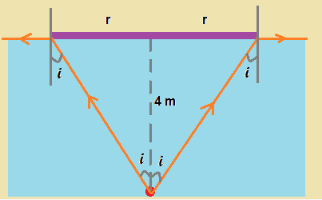We need to block the light rays using the disc till total internal reflection takes place.

As shown in figure, the direction of light ray at the edge of disc is parallel to light surface, when the incident ray makes critical angle
After the edge, light rays will be reflected due to total internal reflection and the whole radiation from light source is blocked

Hence the angle i shown in figure should be critical angle.
For critical angle we have,  sin i = 1/μ = 3/5

hence tan i = 3/4 ....(1)

From figure, we see tan i = r/4  ....(2)

hence from (1) and (2),  the required radius = 3 m
QUESTION: 14

In the following question, a Statement of Assertion (A) is given followed by a corresponding Reason (R) just below it. Read the Statements carefully and mark the correct answer-
Assertion(A): Torque is an axial vector and directed along the axis of rotation.
Reason(R): Torque is equal to vector product of force to the position vector.

Solution:
QUESTION: 15

In order to double the frequency of the fundamental note emitted by a stretched string, the length is reduced to 3/4 th of the original length and the tension is changed. The factor by which the tension is to be changed is

Solution:
QUESTION: 16

Three sound waves of equal amplitudes have frequencies v − 1 , v , v + 1. They superpose to give beats. The number of beats produced per second will be-

Solution:
p1 =po sin 2π(x- 1)t
p2 =po sin 2π(x)t
p3 =po sin 2π(x+ 1)t
p=p1+p3+p2
=posin2π(x-1)t +po sin 2π(x+1)t +po sin 2π(x)t
= 2posin2πxtcos2πt +posin2πxt
= 2posin2πxt[2cos πt +1]
⇒ fbeat = 2
QUESTION: 17

70 calories of heat are required to raise the temperature of 2 moles of an ideal gas at constant pressure from 30oC to 35oC. The amount of heat required to raise the temperature of the same sample of the gas through the same range at constant volume is nearly (Gas constant = 1.99 cal/K-mole)

Solution:
QUESTION: 18

Water is flowing on the blades of a turbine at a rate of 100 kg-s-1 from a certain spring. If the height of the spring be 100 m, then power transferred to the turbine will be

Solution:
Work done = Force X Distance.

From the question :

Force = 100 X 10 = 1000N

Distance = 100m

Work done = 100 X 1000 = 100000Joules

Power = Work done /Time

The time = 1 second.

Power = 100000/1 = 100000 Watts

= 100kW

QUESTION: 19

If the two slits in Young's experiment have width ratio 1:4, the ratio of intensity at maxima and minima in the interference pattern in

Solution: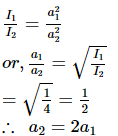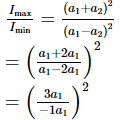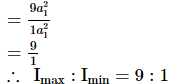QUESTION: 20

Eight negative charges each of value q coulomb are placed at the corners of a cube of side 'a' metre, at the centre of which a positive charge of +2q coulomb is placed.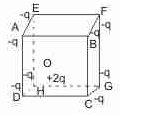Q.
The central charge 2q makes 8 similar pairs with the charges at corners. Their potential energy is :

Solution:
*Answer can only contain numeric values
QUESTION: 21

A small elastic ball of mass m is placed at the apex of a 45° inclined plane as shown in the figure below. The ball is allowed to slip without friction down the plane (along the dotted line), hit the ground (as shown) and bounce along  it. If the height of the inclined plane is h and the coefficient of restitution between the ball and the ground is 0.5, then the distance AB, as marked on the figure, will be N × h, where N is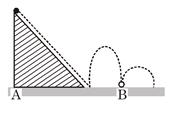Solution: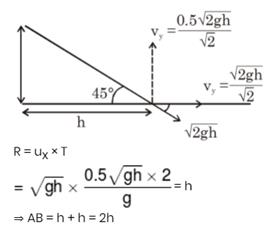*Answer can only contain numeric values
QUESTION: 22

In the circuit in figure we have ε1 = ε2 = 100V, R1 = 20Ω, R2 = 10 Ω, R3 = 40 Ω and R4 = 30 Ω. Find the reading of the ammeter (in A). Disregard the resistance of the battery and the ammeter.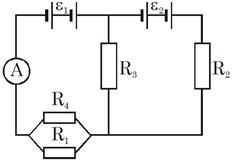Solution: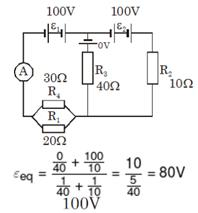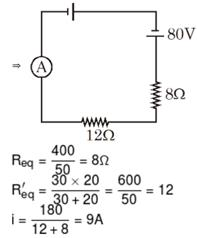*Answer can only contain numeric values
QUESTION: 23

A calorimeter consists of 400 g of water at 24°C. A 500 g piece of copper at 100°C is thrown into the water and the equilibrium temperature is found to be 36.5°C. What is the molar heat capacity of copper in cal/mol k, given that 1 mol of copper has a mass of  63.5g? Neglect the heat capacity of the container.

Solution: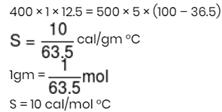*Answer can only contain numeric values
QUESTION: 24

In gravity free space, a bead of charge 1µC and mass 3 mg is threaded on a rough rod of friction coefficient µ = 0.3. A magnetic field of magnitude 0.2 T exists perpendicular to the rod. The bead is projected along the rod with a speed of 4m/s. How much distance (in m) will the bead cover before coming to rest ?

Solution: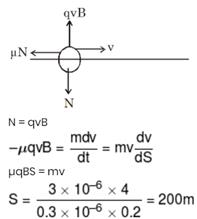*Answer can only contain numeric values
QUESTION: 25

Initially the capacitor is uncharged. What is the steady state charge on it (in µC) after the switch is closed?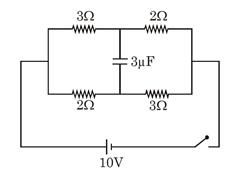Solution: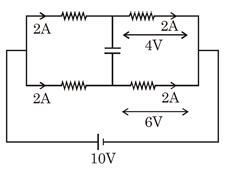Pd = 6 – 4 = 2 V Þ Q = CV = 6µC